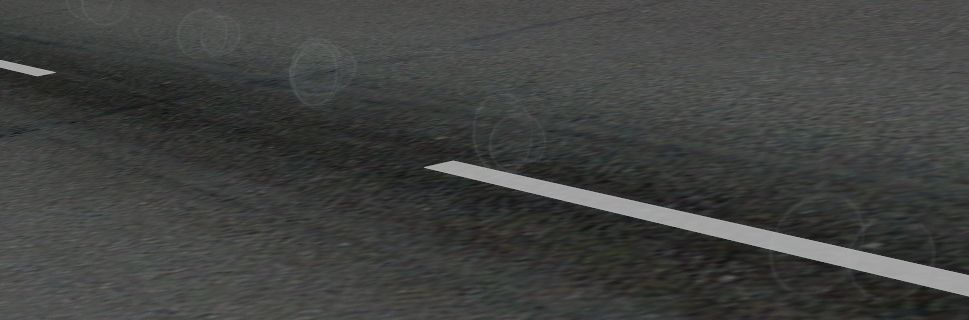# Vortices

#### biggard

Hey there,

I am waaay new to effects editing, so naturally I've worked myself into a corner. I'm working on a little condensation trail effect created by the flaps. I modified a texture to get a circular effect and was hoping to emit several of these per second to create a tube like look.

Here's unfortunately what I ended up with (hopefully you can make it out):What seems to be happening is the effects are being emitted more like puffs (possibly frame rate related even though I have no drops in frames), rather than evenly spaced.

Any idea what might be behind it, or am I approaching this completely the wrong way?

Here's the code:

Code:
``````[Library Effect]
Version=1.00

[Emitter.0]
Delay=0.00, 0.00
Bounce=0.00
Rate=300.00, 300.00
X Emitter Velocity=0.00, 0.00
Y Emitter Velocity=0.00, 0.00
Z Emitter Velocity=0.00, 0.00
Drag=0.00, 0.00
X Particle Velocity=0.00, 0.00
Y Particle Velocity=-5.00, -5.00
Z Particle Velocity=-5.00, -5.00
X Offset=0.00, 0.00
Y Offset=0.00, 0.00
Z Offset=0.00, 0.00

[Particle.0]
Type=19
X Scale=0.10, 0.15
Y Scale=0.10, 0.15
Z Scale=0.10, 0.15
X Scale Rate=0.30, 0.35
Y Scale Rate=0.30, 0.35
Z Scale Rate=0.30, 0.35
Drag=-2.00, -2.00
Color Rate=0.11, 0.11
Rotation=-130.00, 140.00
Face=0, 0, 0

[ParticleAttributes.0]
Blend Mode=1
Texture=dbvap.bmp
Bounce=0.00
Color Start=241, 241, 241, 155
Color End=235, 235, 235, 0
Jitter Distance=0.00
Jitter Time=0.00
TempK=106.80
TempRate=0.00
uv1=0.00, 0.00
uv2=1.00, 1.00
X Scale Goal=0.15
Y Scale Goal=0.15
Z Scale Goal=0.15
Extrude Length=0.00
Extrude Pitch Max=0.00